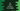# How to find the area of a Triangle in C### How to find the area of a Triangle in C#:

In this article, we will learn how to find the area of a triangle in C#. Finding the area requires the height and the base of the triangle. Once we get both, we can find the area with a simple formula.

It is a beginner-level C# program and with this program, you will learn how to do mathematical calculations, how to take user inputs, and how to print a result back.

### Formula to find the triangle area:

If we know the height and base of a triangle, we can calculate its area by using the below formula:

`area = 1/2 * ( base * height )`

Our program will take the base and height as inputs from the user and calculate the triangle area.

## Algorithm to find the triangle area:

Our program will use the below algorithm to find the area of a triangle:

1. Get the base and height as inputs from the user.
2. Calculate the area by using the above formula.
3. Print the result to the user.

### C# program:

Now, let’s write down the program to do this:

``````using System;

public class Program {
public static void Main() {
float baseSize, height, area;

Console.WriteLine("Enter the base size of the triangle :");

Console.WriteLine("Enter the height of the triangle :");

area = (baseSize * height) / 2;
Console.WriteLine("Area : " + area);
}
}``````

Here,

• baseSize, height, and area are float variables to store the base size, height, and area respectively.
• It is asking the user to enter the base size of the triangle and stores it in baseSize. Console.ReadLine reads the user input as a string. So, we are using float.Parse to parse that value to a floating-point value.
• area is used to store the area that is calculated by using the above formula. The last line is printing the value of the area that is calculated.

### Sample Output:

If you run this program, it will print outputs as like below:

``````Enter the base size of the triangle :
5
Enter the height of the triangle :
5
Area : 12.5

Enter the base size of the triangle :
12.5
Enter the height of the triangle :
13.5
Area : 84.375``````